Sponsored Link •Frequency String function help

1 reply on 1 page. Most recent reply: May 30, 2003 5:24 AM by Rahul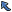Back to Topic List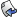Reply to this Topic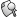Search Forum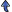Threaded View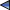Previous Topic Next Topic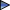Flat View: This topic has 1 reply on 1 page
 jake Posts: 83 Nickname: onorok Registered: May, 2002
Frequency String function help Posted: May 30, 2003 3:32 AMReply
 AdvertisementThis is supposed to go through a String and find the frequency of all the characters in the String, and create a table with the data found.
I am getting out of bounds errors in the getFreq().
Someone else look at this please.

```import java.util.StringTokenizer;
public class HuffmanTree
{
//Some class variables.
char[][] freqTable;
int row=0, col=0;

public HuffmanTree(String sentence){

freqTable=new char[sentence.length()];//2d-array same size as the string arg.
getFreq(sentence, 0);
}
/*****************************************************
Name: getFreq()
Parameters: String, int
Declared variables:char current, bool doIt, int freqCount
char fc.
Return value: void

Algorithm: (assumes the user puts all the characters in the same case)
getFreq(entireString, starting indx 'initialy at 0')
remove white spaces if flag alows.

frequent count=0
current=char @ stIndx
put current in the 2d-frequency array

for(compare every char in the entire string to char @ stIndx)
every true comparison to the char @ stIndx  add one to a
counter for that char.
end for.

remove every instance of the current char.
convert counter int to a char.
put number(counter) in the 2d-frequency array.
++row to drop the place down one.
if(startIndx is not at the end of the string)
recurse.

****************************************************/
public void getFreq(String s, int stIndx){
char current;
boolean doIt=false;

if(doIt){//If doneBefore equals false.
s=removeWhiteSpaces(s);
doIt=true;
}

//while(stIndx!=s.length()+1){
int freqCount=0;
current=s.charAt(stIndx);
freqTable[row][col]=current;

for(int i=0; i<=s.length(); ++i){
if(current==s.charAt(i))
freqCount++;
}

s=removeChar(s,current);//Removes every instance of the current char from sentence.
doIt=false;//Remove all the white spaces again.
char fc=(char)freqCount;

//Adds the frequency to the same row one column over.
fc=freqTable[row];
++row;//Drops down a row, ready for the next one.
//}
if(stIndx<=s.length())//If not at the end
getFreq(s, ++stIndx);//recurse.

}
/*****************************************************
Name: printFreqTable()
Parameters: none.
Declared variables: none except the for loop counters
Return value: void
Purpose: To move through the entire Frequency table and
output it to the screen.

Recursive approach algorithm:
printFreqTable(int startRowIndx, int startColIndx)
print row and column
if col<=col.length
recurse(++startRow, ++startCol)
end.
****************************************************/
public void printFreqTable(){
System.out.println("Frequency Table:");
for(int r=0; r<=freqTable[row].length; ++r){//Print the row.
System.out.println(freqTable[r]+" = ");
for(int c=0; c<=freqTable[col].length; ++c){//Print the column.
System.out.print(freqTable[c]);
}
}
}

public String removeWhiteSpaces(String s){
StringTokenizer sToken=new StringTokenizer(s," ",false);
StringBuffer sbuff=new StringBuffer();
while(sToken.hasMoreTokens()){
sbuff.append(sToken.nextToken());
}
s=sbuff.toString();//Converts the StringBuffer sbuff into a regular String.
return s;
}

//Removes at a specific indx.
public static String removeCharAt(String s, int pos) {
return s.substring(0,pos)+s.substring(pos+1);
}

//Removes all related characters.
public static String removeChar(String s, char c) {
String r = "";
for (int i = 0; i < s.length(); i ++) {
if (s.charAt(i) != c) r += s.charAt(i);
}
return r;
}

//The treeNode innner class.
public class treeNode
{
int frequency;
char value;
char code='0';
boolean isRoot;

public treeNode(int freq, char val, boolean isR)
{
this.frequency=freq;
this.value=val;
this.isRoot=isR;//Set this flag to true if node is root of tree.
}
}

//The treeLeaf inner class.
public class treeLeaf
{
int frequency;
char value;
char code='1';

public treeLeaf(int freq, char val)
{
this.frequency=freq;
this.value=val;
}
}

public static void main(String[] args){
HuffmanTree tree=new HuffmanTree("susie says come over");
//tree.printFreqTable();
}

}
```

thanx.

 Rahul Posts: 52 Nickname: wildhorse Registered: Oct, 2002
Re: Frequency String function help Posted: May 30, 2003 5:24 AMReply
Jake, i did find some errors in the code. but debugging is a pain..so here's what you want (it follows your format so should be easy to understand):

```import java.util.*;

class CharFreq
{
Hashtable ht = new Hashtable();

CharFreq(String s){
s=s.trim();
while (s.length()!=0)
{
int count = 0;
char c = s.charAt(0);
for (int i=0; i< s.length() ;i++ )
{
if (c==s.charAt(i))
count++;
}
ht.put(""+c,new Integer(count));
s = removeChar(s,c);
s=s.trim();
}

display(ht);
}

public String removeChar(String s, char c){
for (int i=0; i< s.length() ;i++ ){
if (i==s.indexOf(c)){
if (i==0){
s = s.substring(i+1,s.length());
}else{
s = s.substring(0,i) + s.substring(i+1,s.length());
}
}
}
return s;
}

public void display(Hashtable ht){
Set st = ht.keySet();
Iterator i = st.iterator();
String temp="";
while (i.hasNext())
{
temp = (String)i.next();
System.out.println(temp+" = "+ (Integer)ht.get(temp));
}
}

public static void main(String[] args)
{
CharFreq cf = new CharFreq("susie says come over");
}
}
```

 Flat View: This topic has 1 reply on 1 pagePrevious Topic Next TopicWeb Artima.com